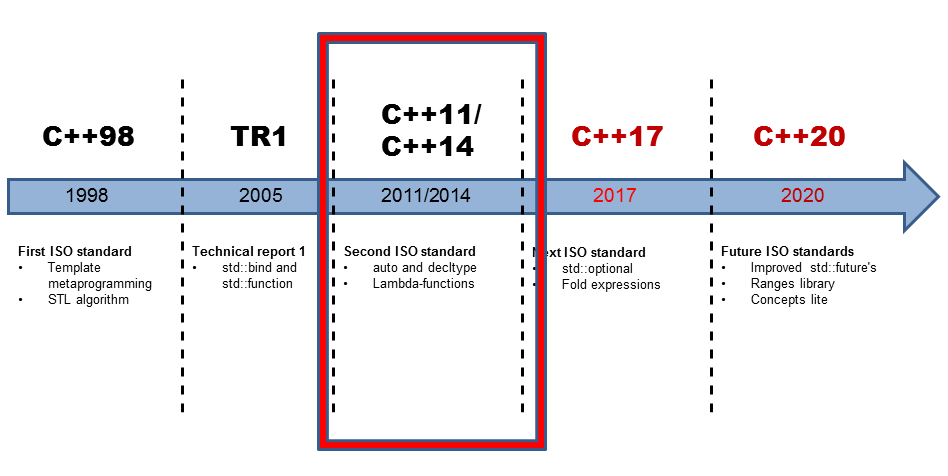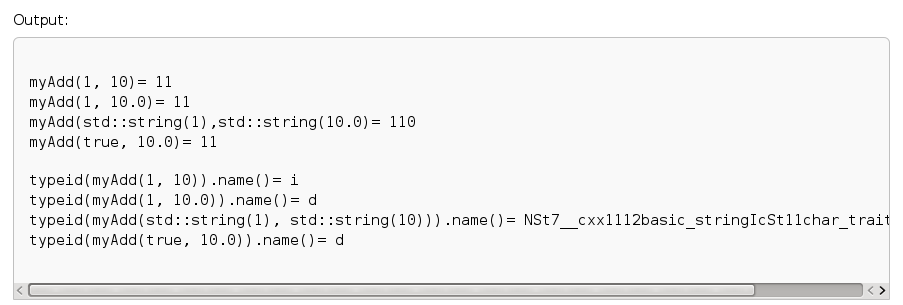# Functional in C++11 and C++14: Dispatch Table and Generic Lambdas

My favorite example, the dispatch table, shows how nicely the features in modern C++ work together. A dispatch table is a table of pointers to functions. In my case, it is a table of handles for polymorphic function wrappers.

But at first, what do I mean by modern C++? I use the dispatch table features from C++11. I added this post, C++14, to the timeline. Why? You will see it later.## Dispatch table

Thanks to Arne Mertz, I used the C++11 features uniform initialization in combination with an initializer list. That further improved the following example.

The example shows a simple dispatch table that maps characters to function objects.

##Modernes C++ Mentoring

Be part of my mentoring programs:

• "Fundamentals for C++ Professionals" (open)
• "Design Patterns and Architectural Patterns with C++" (open)
• "C++20: Get the Details" (reopens December 2023)
• Do you want to stay informed about my mentoring programs: Subscribe via E-Mail.

 ``` 1 2 3 4 5 6 7 8 9 10 11 12 13 14 15 16 17 18 19 20 21 22 23 24 25 26 27 28 29 30 31``` ```// dispatchTable.cpp #include #include #include #include int main(){ std::cout << std::endl; // dispatch table std::map< const char , std::function > dispTable{ {'+',[](double a, double b){ return a + b;} }, {'-',[](double a, double b){ return a - b;} }, {'*',[](double a, double b){ return a * b;} }, {'/',[](double a, double b){ return a / b;} } }; // do the math std::cout << "3.5+4.5= " << dispTable['+'](3.5,4.5) << std::endl; std::cout << "3.5-4.5= " << dispTable['-'](3.5,4.5) << std::endl; std::cout << "3.5*4.5= " << dispTable['*'](3.5,4.5) << std::endl; std::cout << "3.5/4.5= " << dispTable['/'](3.5,4.5) << std::endl; // add a new operation dispTable['^']= [](double a, double b){ return std::pow(a,b);}; std::cout << "3.5^4.5= " << dispTable['^'](3.5,4.5) << std::endl; std::cout << std::endl; }; ```

How does the magic work? The dispatch table is, in my case, a std::map that contains pairs of const char and std::function<double(double, double). Of course, you can use a std::unordered_map instead of a std::map. std::function is a so-called polymorphic function wrapper. Thanks to std::function, it can take all that behaves like a function. This can be a function, a function object, or a lambda function (lines 14 -17). The only requirements of std::function<double(double, double)> are that its entities need two double arguments and return a double argument. The lambda functions fulfill this requirement.

I use the function object in lines 20 – 23. Therefore, the call of dispTable[‘+’] in line 20 returns that function object, which was initialized by the lambda function [](double a, double b){ return a + b; } (line 14). To execute the function object, two arguments are needed. I use them in the expression dispTable[‘+’](3.5, 4.5).

A std::map is a dynamic data structure. Therefore, I can add and use the ‘^’ operation (line 27) at runtime. Here is the calculation.Still, a short explanation is missing. Why is this my favorite example in C++?

### Like in Python

I often give Python seminars. A dispatch table is one of my favorite examples to motivate the easy usage of Python. That is, by the way, the reason why Python needs no case statement.

 ``` 1 2 3 4 5 6 7 8 9 10 11 12 13 14 15 16 17 18 19 20``` ```# dispatchTable.py dispTable={ "+": (lambda x, y: x+y), "-": (lambda x, y: x-y), "*": (lambda x, y: x*y), "/": (lambda x, y: x/y) } print print "3.5+4.5= ", dispTable['+'](3.5, 4.5) print "3.5-4.5= ", dispTable['-'](3.5, 4.5) print "3.5*4.5= ", dispTable['*'](3.5, 4.5) print "3.5/4.5= ", dispTable['/'](3.5, 4.5) dispTable['^']= lambda x, y: pow(x,y) print "3.5^4.5= ", dispTable['^'](3.5, 4.5) print ```

The implementation is based on the functional features of Python. Thanks to std::map, std::function, and lambda-functions, I can now use the same example in C++11 to emphasize the expressive power of C++. A fact I would not have dreamed of ten years ago.## Generic lambda-functions

I almost forgot it. Lambda functions become more potent with C++14. Lambda function can automatically deduce the types of its arguments. The feature is based on automatic type deduction with auto. Of course, lambda functions and automatic type deduction are characteristics of functional programming.

 ``` 1 2 3 4 5 6 7 8 9 10 11 12 13 14 15 16 17 18 19 20 21 22 23 24 25 26 27 28 29``` ```// generalizedLambda.cpp #include #include #include int main(){ std::cout << std::endl; auto myAdd= [](auto fir, auto sec){ return fir+sec; }; std::cout << "myAdd(1, 10)= " << myAdd(1, 10) << std::endl; std::cout << "myAdd(1, 10.0)= " << myAdd(1, 10.0) << std::endl; std::cout << "myAdd(std::string(1),std::string(10.0)= " << myAdd(std::string("1"),std::string("10")) << std::endl; std::cout << "myAdd(true, 10.0)= " << myAdd(true, 10.0) << std::endl; std::cout << std::endl; std::cout << "typeid(myAdd(1, 10)).name()= " << typeid(myAdd(1, 10)).name() << std::endl; std::cout << "typeid(myAdd(1, 10.0)).name()= " << typeid(myAdd(1, 10.0)).name() << std::endl; std::cout << "typeid(myAdd(std::string(1), std::string(10))).name()= " << typeid(myAdd(std::string("1"), std::string("10"))).name() << std::endl; std::cout << "typeid(myAdd(true, 10.0)).name()= " << typeid(myAdd(true, 10.0)).name() << std::endl; std::cout << std::endl; } ```

In line 11, I use the generic lambda function. This function can be invoked with arbitrary types for its arguments fir and second and deduces in addition automatically its return type. To use the lambda function, I gave the lambda function the name myAdd. Line 13 – 17 shows the application of the lambda function. Of course, I’m interested in which type the compiler derives for the return type. For that, I use the typeid operator in lines 21 -25. This operator needs the header <typeinfo>.

The typeid operator is not so reliable. It returns a C string, which depends on the implementation. You have not guaranteed that the C string is different for different types nor that the C string is the same for each program invocation. But for our use case, the typeid operator is reliable enough.

My desktop PC is broken. Therefore I execute the program at cppreference.com.The output shows the different return types. The C string i and d stands for the types int and double. The type of the C++ strings is not so good readable. But you can see that std::string is an alias for std::basic_string.

## What’s next?

In the next post, I will write about the near and distant functional future of C++. With C++17 and C++20, the functional aspect of C++ becomes more powerful.

Thanks a lot to my Patreon Supporters: Matt Braun, Roman Postanciuc, Tobias Zindl, G Prvulovic, Reinhold Dröge, Abernitzke, Frank Grimm, Sakib, Broeserl, António Pina, Sergey Agafyin, Андрей Бурмистров, Jake, GS, Lawton Shoemake, Jozo Leko, John Breland, Venkat Nandam, Jose Francisco, Douglas Tinkham, Kuchlong Kuchlong, Robert Blanch, Truels Wissneth, Kris Kafka, Mario Luoni, Friedrich Huber, lennonli, Pramod Tikare Muralidhara, Peter Ware, Daniel Hufschläger, Alessandro Pezzato, Bob Perry, Satish Vangipuram, Andi Ireland, Richard Ohnemus, Michael Dunsky, Leo Goodstadt, John Wiederhirn, Yacob Cohen-Arazi, Florian Tischler, Robin Furness, Michael Young, Holger Detering, Bernd Mühlhaus, Matthieu Bolt, Stephen Kelley, Kyle Dean, Tusar Palauri, Dmitry Farberov, Juan Dent, George Liao, Daniel Ceperley, Jon T Hess, Stephen Totten, Wolfgang Fütterer, Matthias Grün, Phillip Diekmann, Ben Atakora, Ann Shatoff, Rob North, and Bhavith C Achar.

Thanks, in particular, to Jon Hess, Lakshman, Christian Wittenhorst, Sherhy Pyton, Dendi Suhubdy, Sudhakar Belagurusamy, Richard Sargeant, Rusty Fleming, John Nebel, Mipko, Alicja Kaminska, Slavko Radman, and David Poole.

 My special thanks to EmbarcaderoMy special thanks to PVS-StudioMy special thanks to Tipi.buildMy special thanks to Take Up Code## Seminars

I’m happy to give online seminars or face-to-face seminars worldwide. Please call me if you have any questions.

### Standard Seminars (English/German)

Here is a compilation of my standard seminars. These seminars are only meant to give you a first orientation.

• C++ – The Core Language
• C++ – The Standard Library
• C++ – Compact
• C++11 and C++14
• Concurrency with Modern C++
• Design Pattern and Architectural Pattern with C++
• Embedded Programming with Modern C++
• Generic Programming (Templates) with C++

#### New

• Clean Code with Modern C++
• C++20

### Contact Me

Modernes C++ Mentoring,Tags:
0 replies